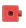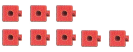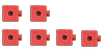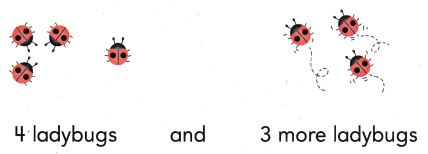Refer to our Texas Go Math Grade 1 Answer Key Pdf to score good marks in the exams. Test yourself by practicing the problems from Texas Go Math Grade 1 Module 4 Assessment Answer Key.

Vocabulary
Underline the sum. (p.122)

Question 1.
3 + 1 = 4Explanation:
Circle the addends and underlined the sum.

Concepts and Skills

Useto show adding to.
Draw the. Write the sum. TEKS 1.3.B, 1.5.E

Question 2.
3 ants and 5 more ants
3 + 5 = ___Explanation:
the sum of 3 and 5 are 8

Question 3.
2 owls and 4 more owls
2 + 4 = ___Explanation:
the sum of 2 and 4 are 6

Draw circles to show the number. Write the sum. TEKS 1.3D, 1.5.G

Question 4.

0 + 5 = ___
0 + 5 = 5
Explanation:
The sum of 0 and 5 is 5
If some number is added with 0 the number will be the answer

Question 5.

6 + 0 = ____
6 + 0 = 6
Explanation:
The sum of 0 and 6 is 6
If some number is added with 0 the number will be the answer

Texas test Prep

Question 6.
Which shows how many? TEKS 1.3.B(A) 7
(B) 4
(C) 3
Explanation:
The sum of 4 and 3 is 7

Question 7.
Which shows the addends in a different order? TEKS 1.3.D, 1.5.G(A) 5 = 4 + 1
(B) 2 + 5 = 7
(C) 4 + 5 = 9
Explanation:
4 + 5 = 9
shows the addends in a different order

Question 8.
Which is a way to make 10? TEKS 1.3.C
(A) 3 + 5
(B) 6 + 3
(C) 3 + 7
Explanation:
the sum of 3 and 7 is 10

Question 9.
There are 3 red books and 3 green books. How many books are there? TEKS 1.3.B, 1.5.D(A) 6
(B) 3
(C) 15
Explanation:
The sum of 3 and 3 is 6

Question 10.
Ella sorts 5 marbles in 2 boxes. Which is a way she can sort the marbles? TEKS 1.3.C
(A) 3 + 5 = 8
(B) 6 + 3 = 9
(C) 3 + 7 = 10